## python数据分析及展示（二）

1. Matplotlib库的介绍

http://matplotlib.org/gallery.html可查看Matplotlib库的效果

Matplotlib库由各种可视化类构成，内部结构复杂，受Matlab启发
matplotlib.pyplot是绘制各类可视化图形的命令子库，相当于快捷方式

import matplotlib.pyplot as plt

plt.plot([3,1,4,5,2])

plt.plot()只有一个输入列表或数组时，参数被当作Y轴，X轴以索引自动生成

plt.savefig('test',dpi=600)

plt.savefig()将输出图形存储为文件，默认PNG格式，可以通过dpi修改输出质量

plt.plot([0,2,4,6,8],[3,1,4,5,2])

plt.plot(x,y)当有两个以上参数时，按照X轴和Y轴顺序绘制数据点

plt.subplot(nrows, ncols, plot_number)

plt.subplot(3,2,4)或plt.subplot(324)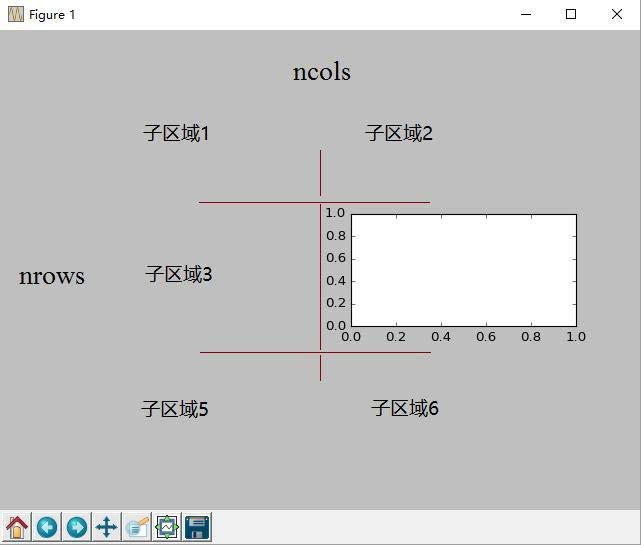2. pyplot的plot()函数

plt.plot(x, y, format_string, **kwargs)
∙ x : X轴数据，列表或数组，可选
∙ y : Y轴数据，列表或数组
∙ format_string: 控制曲线的格式字符串，可选，由颜色字符、风格字符和标记字符组成
∙ **kwargs : 第二组或更多(x,y,format_string)

'b'                 蓝色            'm'             洋红色magenta
'g'                 绿色             'y'             黄色
'r'                  红色             'k'             黑色
'c'                 青绿色cyan  'w'             白色
'#008000'      RGB某颜色 '0.8'          灰度值字符串

'‐'               实线
'‐‐'           破折线
'‐.'             点划线
':'                 虚线
'' ' '               无线条

'.'                  点标记
','                  像素标记(极小点)
'o'                 实心圈标记
'v'                 倒三角标记
'^'                 上三角标记
'>'                 右三角标记
'<'                 左三角标记

'1'                下花三角标记
'2'                上花三角标记
'3'                左花三角标记
'4'                右花三角标记
's'                实心方形标记
'p'                实心五角标记
'*'                 星形标记

'h'                竖六边形标记
'H'                横六边形标记
'+'                十字标记
'x'                x标记
'D'               菱形标记
'd'                瘦菱形标记
'|'                 垂直线标记

color : 控制颜色, color='green'
linestyle : 线条风格, linestyle='dashed'
marker : 标记风格, marker='o'
markerfacecolor: 标记颜色, markerfacecolor='blue'
markersize : 标记尺寸, markersize=20
……

3. pyplot的中文显示

（1）pyplot并不默认支持中文显示，需要rcParams修改字体实现

import matplotlib.pyplot as plt

import matplotlib

matplotlib.rcParams['font.family']='SimHei'

'font.family'           用于显示字体的名字
'font.style'             字体风格，正常'normal'或斜体'italic'
'font.size'              字体大小，整数字号或者'large'、'x‐small'

plt.xlabel('横向：时间',fontproperties='SimHei', fontsize=20)

4. pyplot的文本显示

plt.xlabel()           对X轴增加文本标签
plt.ylabel()           对Y轴增加文本标签
plt.title()              对图形整体增加文本标签
plt.text()              在任意位置增加文本
plt.annotate()      在图形中增加带箭头的注解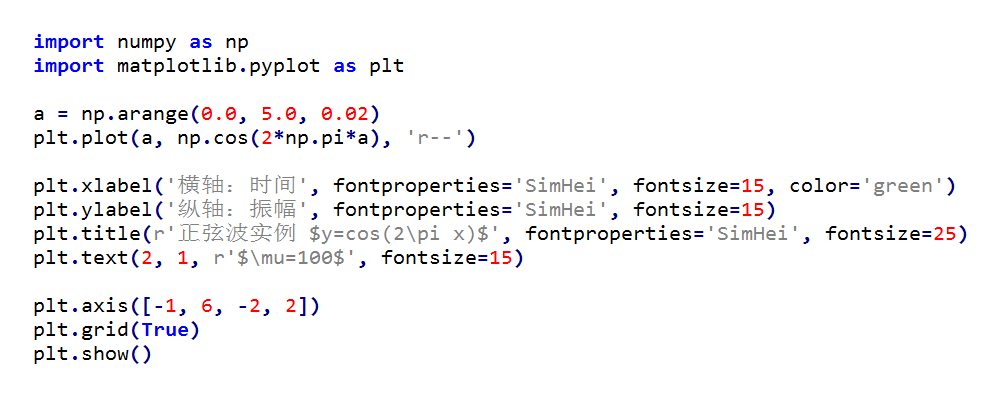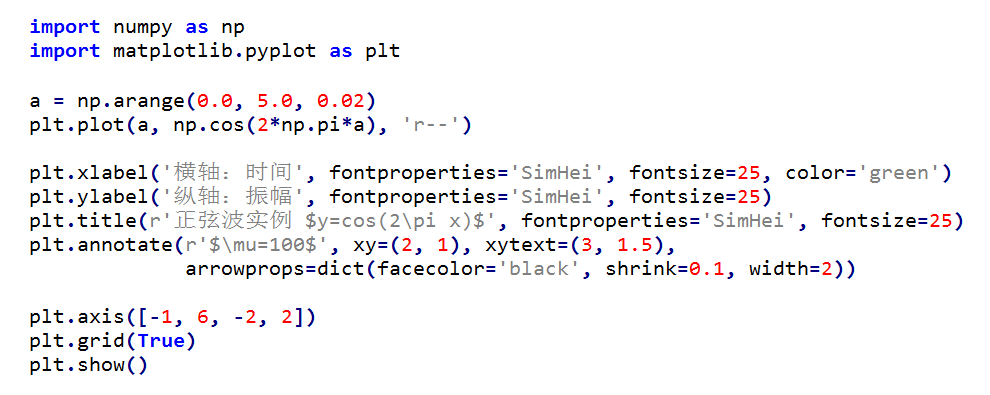5. pyplot的子绘图区域

plt.subplot2grid(GridSpec, CurSpec, colspan=1, rowspan=1)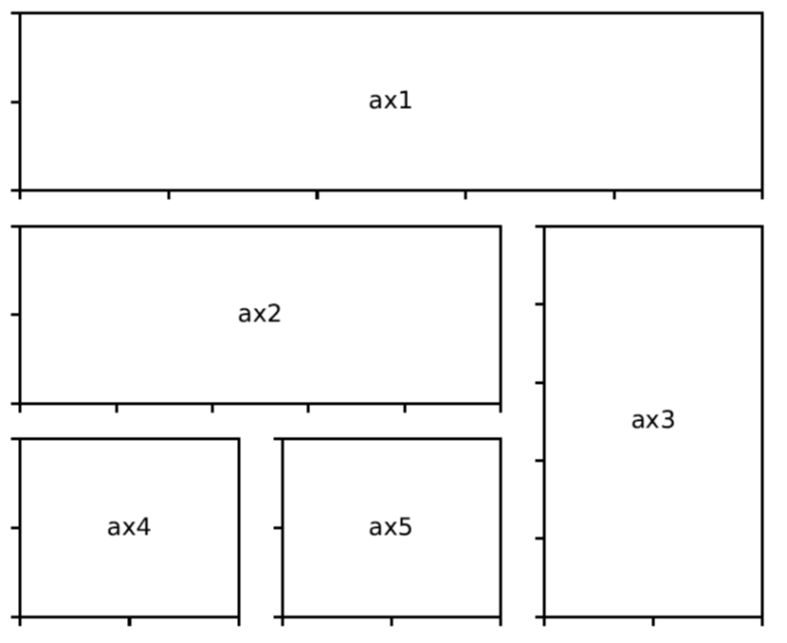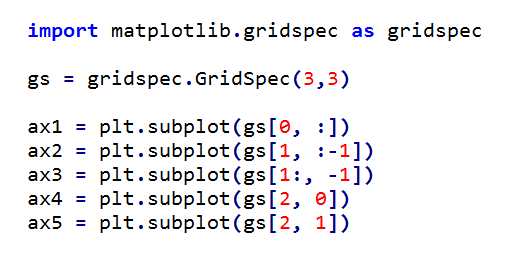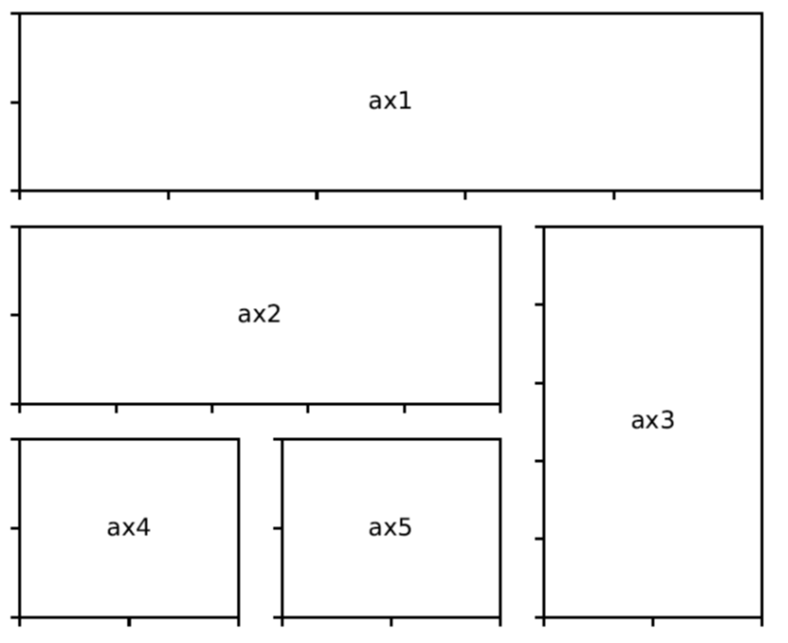1. pyplot基础图表函数概述

plt.plot(x,y,fmt,…)                          绘制一个坐标图
plt.boxplot(data,notch,position)     绘制一个箱形图
plt.bar(left,height,width,bottom)    绘制一个条形图
plt.barh(width,bottom,left,height)  绘制一个横向条形图
plt.polar(theta, r)                           绘制极坐标图
plt.pie(data, explode)                    绘制饼图

plt.cohere(x,y,NFFT=256,Fs)       绘制X‐Y的相关性函数
plt.scatter(x,y)                              绘制散点图，其中，x和y长度相同
plt.step(x,y,where)                       绘制步阶图
plt.hist(x,bins,normed)                 绘制直方图

plt.contour(X,Y,Z,N)                     绘制等值图
plt.vlines()                                    绘制垂直图
plt.stem(x,y,linefmt,markerfmt)    绘制柴火图
plt.plot_date()                              绘制数据日期

2. pyplot饼图的绘制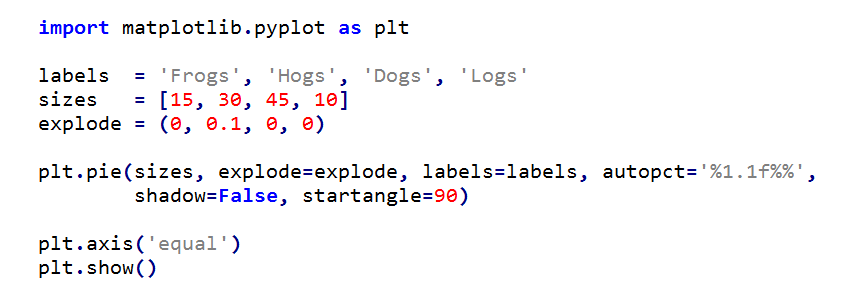3. pyplot直方图的绘制hist 中20表示直方的个数

4. pyplot极坐标的绘制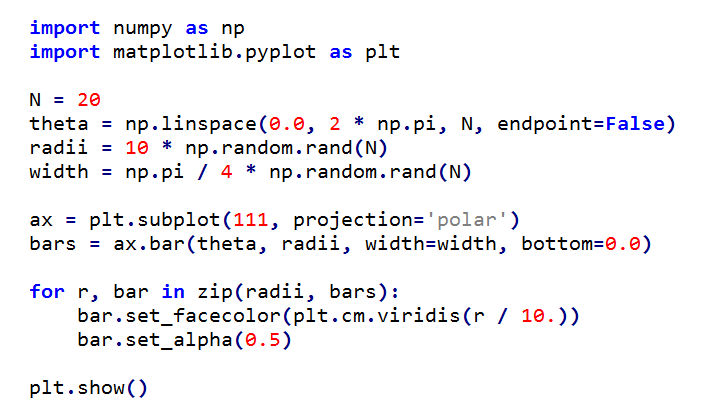5. pyplot散点图的绘制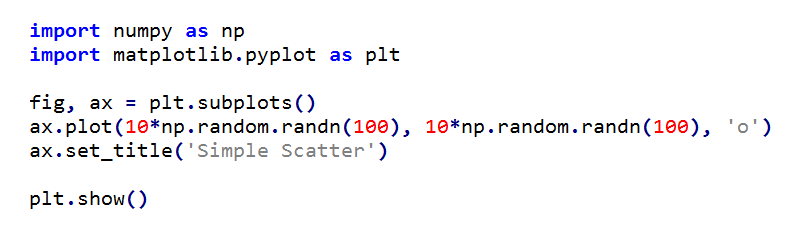posted on 2018-03-12 21:52  n哖苡逅  阅读(579)  评论(0编辑  收藏  举报